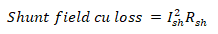# Losses in Electrical Machines – Formulas and Equations

## Losses in Machines Equations and Formulas

The following formulas and equations can be used to calculate and measure the different kinds of losses in an electrical machine such as eddy current and hysteresis as iron core losses and armature and field losses as copper losses.### Copper Losses:

#### Armature Loss:

Armature Cu Losses = Pa = IaRa

Where

#### Field Loss:

Field Cu Losses = Pf = If2Rf

Where

• If is the field current
• Rf is the field resistance

For Shunt Field:Where

• sh is the shunt field current
• sh is the shunt field resistance

For Series Field:Where

• se is the series field current
• se is the series field resistance

Related Posts:

### Iron/Core Losses

#### Hysteresis Loss:Where

• η = hysteresis or Steinmetz’s constant
• Bmax = maximum value of the magnetic flux density
• f = frequency of magnetization
• V= volume of the core

AlsoWhere

• P is the number of poles
• N is the speed in RPM

#### Eddy Current Loss:or
We = PB2max f2t2   Watts

Where

• is the electrical constant of the core material
• Bmax is the maximum flux density
• f is the frequency of magnetization
• t is the thickness of lamination
• V is the volume of the core

### Brush Losses in DC Machine:

PBD = VBD x Ia

Where:

• PBD = Power loss due to brush drop
• VBD = Voltage drop due to brush drop
• Ia = Armature Current

Other machinal loss and stray loss in a machine depends on multiple factors and different for each case and machine such as transformer, motor, alternator etc.

Related Formulas and Equations Posts: